# Validity, Formula, Graph

A function is a set of ordered pairs whereby for each element in the first place (domain) there is exactly one element in the second place (range).

When defining a function, it is necessary to consider what values are possible for the domain, in the context of the the purpose of the function.

In a real life context, we take data values, plot them and consider what kind of mathematical relation is similar to the data values. (The project). This is called modelling.

In a mathematical context, we are often able to calculate an algebraic relationship between the domain and the range.

# Example: Large and Small Squares

Consider this square that measures 8 cm by 8 cm and has a smaller square of side lengthcm cut out of it. How much area is remaining?

The area remaining depends on the value of.

Let’s create a function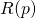that maps the value ofto the area remaining, that is,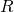.

# Validity

First, we need to consider what values ofare possible in this context.

For a square to exist, we need thatis bigger than (or equal to) zero. We can make sense of ‘no square’ but we can’t make sense, in the context of paper and scissors, of a square that has a negative side length.

We also require that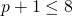.

Therefore: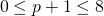in other words,# Ordered pairs

Second, let’s calculate some ordered pairs that are part of this function.

When, the side length of the small square is, nowso the area remaining is.

When, the side length of the small square is, nowso the area remaining is.

When, the side length of the small square is, now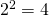so the area remaining is.

and so on….

We can plot the points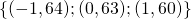on a graph.

# Formula

Finally, since we are repeating the same calculation, let’s write it down in general:

We begin with area 64, and subtract the area of the small square.

The area of the small square =Therefore, our formula is:To write it accurately, we should say:The graph (click on graph a few times):

To create this graph on GeoGebra, type in or copy/paste: R(p)=63-2p-p^2, -1<=p<=7 to the input bar. To plot the points, you may plot them individually, or you may plot them as a sequence: Sequence[(p,63-2p-p^2),p,-1,7]

# Corner Cut Off

A rectangle is made of blue paper and measures 20 cm by 10 cm.

A corner is cut off.

The corner that is cut off is a right angled triangle, with shorter sidescm andcm.

What values ofare valid?

Create a formulathat maps the value p on the slider to the value Hypotenuse.

Create a formulathat maps the value p on the slider to the value Area Remaining.

Check both formula using the value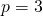.

Graph your functionand your functionon Geogebra for the valid values of.

Solution:

# Four Corners Cut Off

Four identical squares are cut from the corners of a piece of blue paper measuring 8 cm by 8 cm.

The side length of one the squares cut off iscm.

What are the valid values of?

Create a function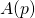that maps the value of p shown on the slider to the Area Remaining, remember to state the domain with the function.

Graph the function for values ofin the domain. State the range of the function.

# Rectangle in Semi Circle

A rectangle is drawn inside a semi circle that has radius 5 cm.

Find a function that maps the valueon the slider to the area of the rectangle.

Graph the function for valid values of. State the domain and the range of the function.

# Inscribed Polygon

A regular polygon with p sides is drawn inside a circle with radius 5 as shown.

Show through calculation that the area of the polygon, when p = 7, is 68.41.

Calculate a functionthat maps the valueto the area of the polygon.